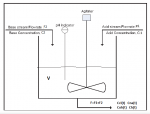# pH Neutralization Process - Obtaining transfer function

#### Alexpaok44

Hello, I am at my final year of MSc in Electrical engineering and my thesis is about control in a pH neutralization process.

The aim is to control the pH of the system and the chemical equation which occurs in the CSTR is: HCl + NaOH->H + OH + Na + Cl

The input variables of the system are:
-F1 ( HCL flow rate ) [500 lt/min]
-F2(t) ( NaOH flow rate )
-C1 ( inlet concentration of HCl ) [0.01mol/lt]
-C2 (inlet concentration of NaOH ) [0.1mol/lt]

The output variables are:
-F=F1+F2(t) (total flow rate at the exit)
-Ccl(t) (outlet concentration of Cl ions )
-Cna(t) (outlet concentration of Na ions)
-Ch(t) (outlet concentration of Hydrogen ions)
-Coh(t) (outlet concentration of OH ions)

The constants are:
V : volume of CSTR [20000lt]
Kw : water dissociation constant [1e-14 mol^2/lt^2]The equations that take place here are:
i)Electroneutrality: Ccl+Coh=Cna+Ch
ii)pH definition: pH=-log10(Ch)
iii)Coh*Ch=Kw
iv)mass balances: V*dCcl/dt=F1*C1-(F1+F2)*Ccl V*dCna/dt=F2*C2-(F1+F2)*Cna

The pH of the system is considered to be the output of the system and F2 is considered to be the input (u).Everything else is considered to be constant.

I have managed to represent this in state space form and perform successfully I/O feedback linearization.Specifically:
if x1=Ccl and x2=Cna and x=x1-x2=Ccl -Cna=Ch-Coh
then the system is : V*dx/dt=F1*C1-F2*C2-(F1+F2)*x or in terms of y,x,u: V*dx/dt=F1*C1-u*C2-(F1+u)*x
Also, Ch=[x+sqrt(x^2+Kw)]/2 and the output y=pH=-log10(Ch)

But I have noticed that a lot of papers obtain a transfer function of the system even though it is nonlinear.
Does anyone have any idea how to accomplish that?
I have failed to linearize it around an operating point and obtain the desirable transfer function-> pH(s)/Fb(s)
Some papers use a method called First Order plus Time Delay Model (or FOPTD) but I have found zero theory over that method.

Any ideas or suggestions would be highly appreciated.Thank you in advance!